## Forum Posts

mr.ricklu
Sep 13, 2021
In Java for ACSL
No homework for this week because we need time to figure out repl.it. Here are the slideshows though: Course overview: https://docs.google.com/presentation/d/1-JOPp45VfS0adZ3CdmWeWoVqmVtoaleE9yvd7zr01Bw/edit?usp=sharing Lesson: https://docs.google.com/presentation/d/1vPB5I9h4OWOHG28T8JuZUSHwSKjvZZqktLnJV1q8r4M/edit?usp=sharing
1
0
36
mr.ricklu
Aug 05, 2021
In CS Fundamentals for ACSL
Which nodes have the MOST paths of length 3 between them?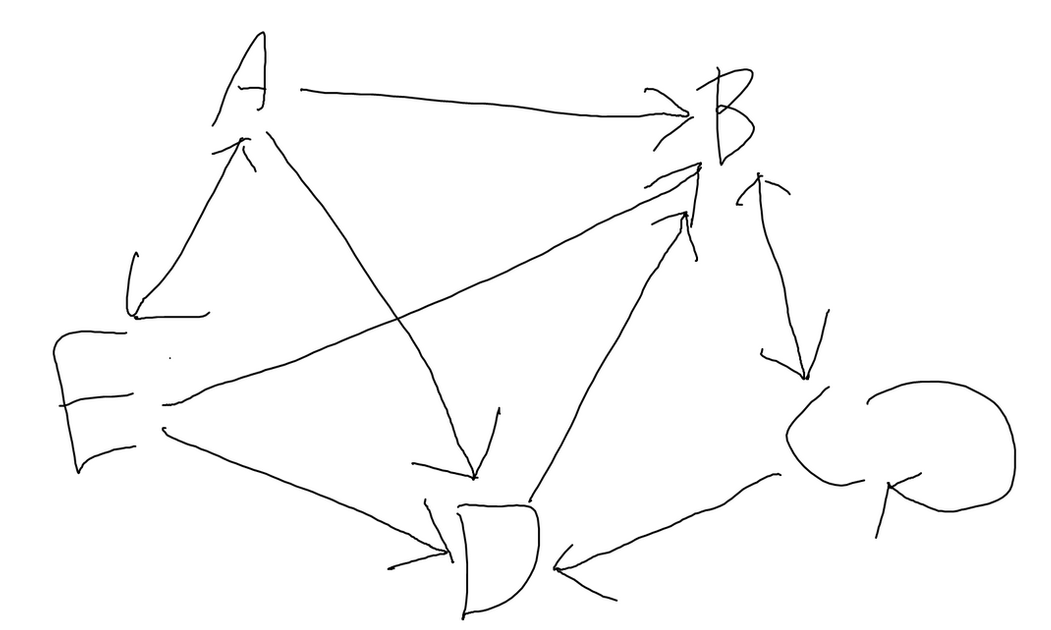0
0
17
mr.ricklu
Aug 02, 2021
In CS Fundamentals for ACSL
What are all the paths of length 3 from E to B in this graph?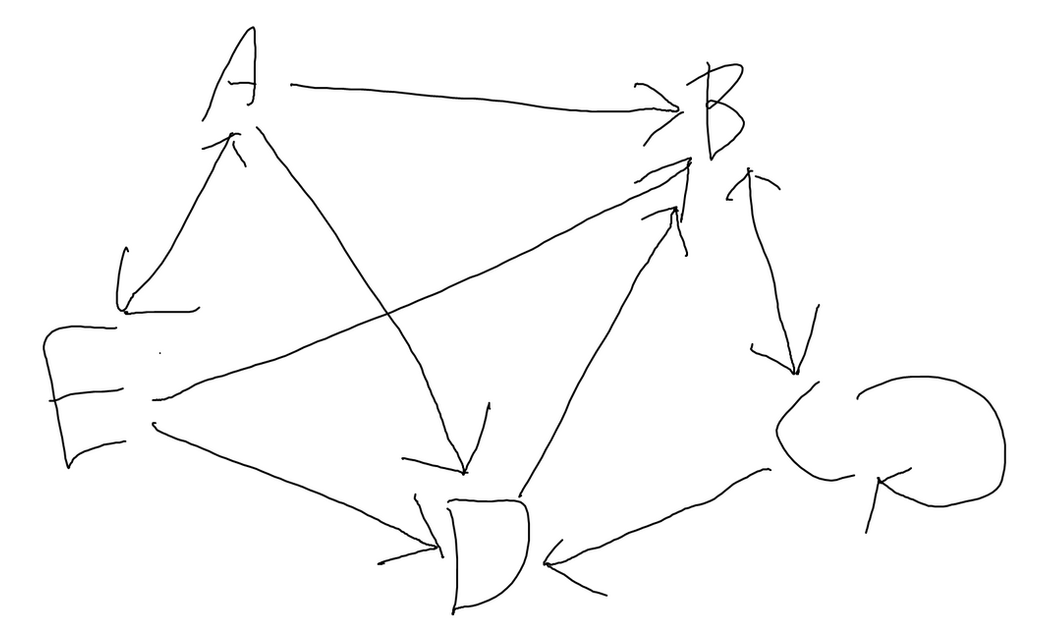0
4
27
mr.ricklu
Jul 25, 2021
In CS Fundamentals for ACSL
Evaluate the next item popped in this sequence if it were a queue, and then if it were a stack: PUSH(C) POP(X) PUSH(U) PUSH(Y) POP(X) PUSH(X) PUSH(H) PUSH(G) PUSH(A) PUSH(Q) POP(X) POP(X) PUSH(N) PUSH(L) POP(X) PUSH(E) PUSH(F) POP(X) POP(X)
0
3
30
mr.ricklu
Jul 22, 2021
In CS Fundamentals for ACSL
Start out with an empty list of lists. What are the contents of row 2 after these operations? BONUS: Instead of using a 2D array, it is possible, though more complicated, to use a 1D array instead. For example, this would be how to convert a 2x2 array to a 1D array with 4 elements: Given the index of an element of the 1D array, how can you convert it into the indices for the same element in the 2D array? For example, an index of 1 would be come (0,1) in the 2D array.0
4
32
mr.ricklu
Jul 11, 2021
In CS Fundamentals for ACSL
Simplify these boolean expressions (You can use ! and brackets to represent NOT): How many outputs are true for the following expression?: If you have forgotten anything, this page has a list of truth tables and laws: http://www.categories.acsl.org/wiki/index.php?title=Boolean_Algebra0
6
67
mr.ricklu
Jun 22, 2021
In CS Fundamentals for ACSL
In case anybody is still confused about logic operators, more information can be found here: http://www.categories.acsl.org/wiki/index.php?title=Bit-String_Flicking (or you can look it up yourself). I've also attached a table of what each operation outputs for reference. HW Questions (Do stuff in brackets first!): LSHIFT-2(10011 XOR 00110) OR (LCIRC-2(RCIRC-2(10001))) ( (RSHIFT-3(01000) XOR NOT(00110) ) AND (01101 OR RCIRC-2(10011)) BONUS: What should X be to satisfy the following equation? (There are multiple answers; name as many as you want!) X AND 11010 = 01000
0
7
59
mr.ricklu
Jun 18, 2021
In CS Fundamentals for ACSL
What are the results of these binary equations? Express your answer in binary as well. 11010110 + 1101101 = 11010000 – 10110011 = 1010110 × 110 = 10011010 ÷ 111 = What is the result of this mixed equation? The numbers in square brackets indicate the base of the preceding number. Express your answer in decimal. 10010 * 2 ÷ (CC + 5 - 315) =
0
7
58
mr.ricklu
Jun 14, 2021
In CS Fundamentals for ACSL
1. Convert the number 319 (in decimal) to binary, octal, and hexadecimal 2. Convert these numbers to decimal: 100011000 (in binary), 1040 (in octal), and AF5 (in hexadecimal) BONUS: What is 10110 (in binary) multiplied by 66 (in octal)? Express your answer in decimal. If you forgot any of the concepts, you can use this website as a refresher: http://www.categories.acsl.org/wiki/index.php?title=Computer_Number_Systems.
0
10
77
mr.ricklu
May 24, 2021
In CS Fundamentals for ACSL
The mock contest: https://docs.google.com/forms/d/e/1FAIpQLSdKgjK0YsyCQPQtPFigO0txMcFzzh-Ltes8R1pWo33T-AbPdg/viewform?usp=sf_link Boolean Algebra: Bit-String Flicking: Recursive Functions: Digital Electronics: Prefix-Infix-Postfix Computer Number Systems: What Does This Program Do: Data Structures: Graph Theory:0
0
14
mr.ricklu
May 24, 2021
In CS Fundamentals for ACSL
You've completed the last class of this course! The finals contest is on Saturday so here is some material to help review. Do as much as you think you need. If you have forgotten anything, this website can help you review: http://www.categories.acsl.org/wiki/index.php?title=Main_Page. Boolean Algebra: Bit-String Flicking: Let X = abcde. Solve the following bit string equation for X: (LCIRC-3 (RSHIFT-1 X) OR 11010 AND NOT (RCIRC-2 10001)) = ((LSHIFT-2 10101) XOR 01110) Solve for X (5-bits) in the following equation: NOT X AND (LSHIFT-1(RCIRC-2 01011)) = 10000 What is the final value of X? X = 11010011010 FOR k = 1 TO 71 X = (RCIRC-k X) NEXT k Recursive Functions: Digital Electronics: Prefix-Infix-Postfix: Given the following operations: % means MOD (integer remainder) such that 5%2 = 1 and \ means DIV (integer quotient) such that 5\3 = 1, evaluate the following prefix expressions if A=5, B=12, C=8, and D=20. Note that all numbers in the original expression are single digits. / - ^ \ * C - - D A B A \ ^ % + A C - D B 2 7 1 - B % B A The trinary operator diff takes 3 arguments a, b, and c and returns the maximum of abs(a-b), abs(b-c), and abs(a-c), where abs(x) is the absolute value of x. Evaluate the following prefix expression: diff / 8 2 diff + 2 1 – 4 3 ↑ 2 3 * 2 3 Define the following unary operators as: # = largest prime number less than a & = smallest prime number greater than a Evaluate the following prefix expression: − / * + # 3 & 6 # 12 # 4 / / * 4 + / 6 # 3 & 2 # 3 & 2 Computer Number Systems: Tickets for a concert were numbered from 1 16 to AA 16 . If when the ticket number is converted to binary it contains the string “10101”, then the lucky ticket holders will receive a Back Stage Pass for after the concert. How many (one pass per winning ticket) passes were given out if the concert was sold out? The ACSL hardware engineers have recently developed a 5-bit memory chip using radical new biotechnology. Unfortunately, the chip melts whenever a value containing the pattern &quot;101&quot; appears within the 5 bits. Of the 32 possible values that the chip can hold, how many will cause the chip to melt? How many of the binary representations of the numbers from 2 to 2047, inclusive, are palindromes? (Lead digit cannot be 0.) What Does This Program Do?: Data Structures: Given the binary search tree for LOSANGELESCA, delete the root node. List the nodes at depth 3 from left to right. Using the ACSL definition, build a heap from the keys N E W H A M P S H I R E (in that order). List the nodes at depth 2, from left to right. Given an initially empty stack and the string DISNEYWORLDORLANDOFLORIDA, perform the following: pop if it is a vowel and push if it is a consonant. If the next string added is a vowel, what item would be popped? Vowels are A, E, I, O, U. Graph Theory: In a directed graph, the paths represent streets from one intersection to another. The intersections are: {A, B, C, D, E, F, G}. The streets are: {AB, BF, EF, CD, ED, DC, EB, FA, GF, FE, BE, CE, GA, FG, AG, FB}. A new one-way street was added from B to C and the two-way street between F and G was closed due to non-use. What was the change in the number of cycles from A between the first directed graph and the second one? Answers: Boolean Algebra: ABC (0, 0, 1), (1, 0, 0) Bit-String Flicking: *0*1* 0*1** 10101101001 Recursive Functions: 11 15 -15 Digital Electronics: 1 4 12 Prefix-Infix-Postfix: 6.3 3 29 Computer Number Systems: 15 11 93 What Does This Program Do?: 90 40 II,III,V Data Structures: E,O H, H, M, P F Graph Theory: 2 fewer 0 110
0
41
mr.ricklu
May 17, 2021
In CS Fundamentals for ACSL
What does this program do: Continue the programming problem from last time: Sample input: 2, 5, 3, 5, 4, 4, 3 3, 4, 5, 3, 2, 2, 1 2, 1, 2, 4, 5 1 2 3 4 5 Sample output: 1 0 0 3 3 Test input: 1, 1, 2, 4, 3, 4, 1 2, 3, 1, 5, 4, 2, 4 1, 2, 4, 2, 1 2 5 1 2 4 Test output: 3 1 0 0 0 ANSWERS: What does this program do: 55. The three arrays look like this after the program has finished (notice the patterns! Almost every question like this has a pattern and it helps speed things up): Programming (sorry for any formatting issues): My python solution: def remove(hand): count = [0,0,0,0,0] for i in hand: count[i-1]+=1 for i in range(5): if count[i]>=4: while i+1 in hand: hand.remove(i+1) return hand opp = input().split(", ") pla = input().split(", ") dec = input().split(", ") oppo = [] play = [] deck = [] for i in range(7): oppo.append(int(opp[i])) play.append(int(pla[i])) for i in range(5): deck.append(int(dec[i])) for i in range(5): num = int(input()) if num in oppo: while num in oppo: oppo.remove(num) play.append(num) else: play.append(deck) del deck play = remove(play) count = 0 for i in play: if i==num: count += 1 print("output:",count) My java solution: import java.util.*; class Main { public static ArrayList<Integer> remove(ArrayList<Integer> hand){ int[] count = new int; for(int i : hand){ count[i-1]++; } for(int i=0; i<5; i++){ if(count[i]>=4){ for(int j=0; j<hand.size(); j++){ if(hand.get(j)==i+1){ hand.remove(j); j--; } } } } return hand; } public static void main(String[] args) { Scanner in = new Scanner(System.in); String[] opp = in.nextLine().split(", "); String[] pla = in.nextLine().split(", "); String[] dec = in.nextLine().split(", "); ArrayList<Integer> oppo = new ArrayList<Integer>(); ArrayList<Integer> play = new ArrayList<Integer>(); ArrayList<Integer> deck = new ArrayList<Integer>(); for(int i=0; i<7; i++){ oppo.add(Integer.parseInt(opp[i])); play.add(Integer.parseInt(pla[i])); } for(int i=0; i<5; i++){ deck.add(Integer.parseInt(dec[i])); } for(int i=0; i<5; i++){ int num = in.nextInt(); if(oppo.contains(num)){ for(int j=0; j<oppo.size(); j++){ if(oppo.get(j)==num){ oppo.remove(j); play.add(num); j--; } } } else { play.add(deck.get(0)); deck.remove(0); } play = remove(play); int count = 0; for(int j : play){ if(j==num){ count++; } } System.out.println(count); } } }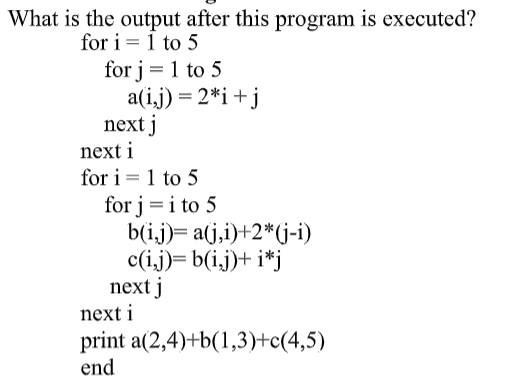0
1
27
mr.ricklu
May 12, 2021
In CS Fundamentals for ACSL
Define REV(X) to mean reverse the elements in the data structure and SWITCH(X) to mean switch the data structure from a stack to a queue or a queue to a stack. What is popped next if it starts as a queue?: PUSH(F), POP(X), PUSH(B), PUSH(J), PUSH(A), PUSH(T), SWITCH(X), PUSH(O), PUSH(P), POP(X), POP(X), POP(X), PUSH(L), PUSH(F), REV(X), PUSH(R), PUSH(B), POP(X), PUSH(V), PUSH(N), PUSH(E), POP(X), PUSH(I), SWITCH(X), POP(X), POP(X), PUSH(U), PUSH(S), POP(X), REV(X), POP(X), POP(X), POP(X), PUSH(Q), POP(X), POP(X), SWITCH(X), PUSH(D), POP(X), POP(X), PUSH(H), PUSH(J), SWITCH(X), PUSH(C), REV(X), PUSH(W), PUSH(G), POP(X) Make a BST of the word PERIODICTABLE, then delete D, delete T, and delete A. What is the internal path length (sum of depths of every node) of the resulting tree? Make a heap of the word CARRYON, then delete the top node twice. How many external nodes are in the resulting tree? In case you forgot anything, here is a website with a review of what we learned: http://www.categories.acsl.org/wiki/index.php?title=Data_Structures
0
1
11
mr.ricklu
May 05, 2021
In CS Fundamentals for ACSL
Prefix/postfix: Evaluate this postfix expression if a=3, b=-2, and c=5: a b - c / b ^ a - c 2 a * 3 b * - * + Define a%b to be 3a+7b, evaluate this prefix expression: % - + 3 1 % 3 4 / 6 + 1 + 1 1 Define a#b to be the maximum of a/b and b/a, and a!b to be 2a-b, evaluate this postfix expression: 6 3 ! 2 1 / + 3 - 9 3 # 2 - + 8 8 2 + + # Digital electronics: Rectangle takes in 3 inputs and is true if exactly 1 input is true. Oval takes in 3 inputs and is true if all 3 inputs are true. How many ordered quadruples make the following circuit false? 2. Square takes in 3 inputs and is true if no inputs are true. X takes in 3 inputs and is false if exactly 2 inputs are true. How many ordered quadruples make the following circuit true?0
1
15
mr.ricklu
Apr 26, 2021
In CS Fundamentals for ACSL
Graph theory: 1. How many paths of length 3 are there that end at D? 2. How many cycles are in this graph? Bit-string flicking: Solve for X: RCIRC-4(LSHIFT-1(X OR 00101) AND RCIRC-2(11011 AND 10111)) = 11001 AND 11100 OR LSHIFT-3(01111 AND 11011) Solve for X: LSHIFT-1(00101 OR RCIRC-2(X XOR 01010)) = LSHIFT-1(11010 XOR 00111) RCIRC-3(01101 XOR (X AND 10110)) = 01101 Solve for X: RSHIFT-2(00101 OR 00011 AND RCIRC-2(01011)) = LCIRC-2(01110 AND RCIRC-4(00100 OR X))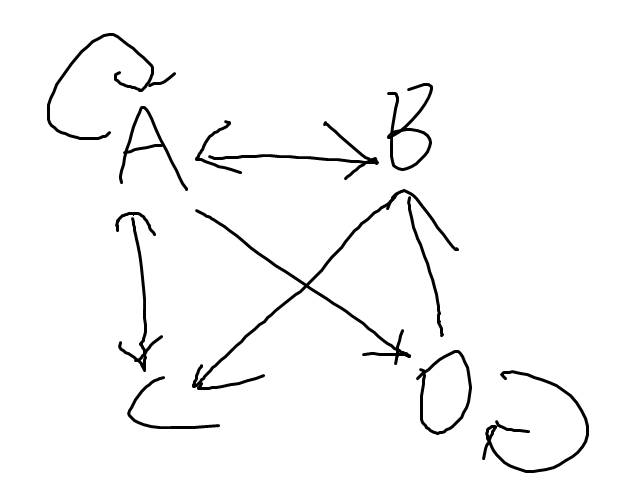0
1
11
mr.ricklu
Apr 19, 2021
In CS Fundamentals for ACSL
Computer number systems: 1. How many times does "010" appear in the binary representations of the octal numbers 12 to 71 inclusive? (leading 0s not included) 2. How many total 0s are in the binary representations of the hex numbers E to 20 inclusive? 3. How many 9-bit binary numbers are palindromes? (no leading 0s) 4. The number in brackets indicates the base. Complete the sequence: 10(10), 17(16), 100100(2), 61(8), ? (10) Boolean Algebra: 5. Simplify: AC+!(B XOR A)+!B 6. Simplify: !(AB+ B XOR C) !C 7. Simplify: C XOR C 8. How many inputs are TRUE for the following expression: A(B XOR C)+!A!(B XOR C)
0
1
16
mr.ricklu
Apr 11, 2021
In CS Fundamentals for ACSL
I'll post the remaining 5 problems from other topics as homework. How many 1’s are there in the binary representation of this octal number: 16743 Evaluate: (LCIRC–2 (RSHIFT–1 (RCIRC–2 (LSHIFT–2 10101)))) Given an initially empty stack and the following sequence of operations, what would be the next POPPED element? PUSH(O), PUSH(C), PUSH(E), POP(X), PUSH(A), PUSH(N), POP(X), POP(X), PUSH(S), PUSH(T), POP(X), PUSH(A), PUSH(T), PUSH(E), POP(X), POP(X) Simplify this digital circuit: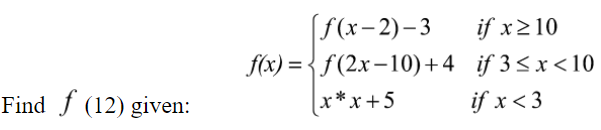0
2
19
mr.ricklu
Mar 22, 2021
In CS Fundamentals for ACSL
Digital Electronics: Here is a website with all the logic gates in case you forgot any: http://www.categories.acsl.org/wiki/index.php?title=Digital_Electronics Simplify these digital circuits: How many outputs are FALSE for these expressions? Answers: !A+B+!C+D !A!B!C !A+!B 4 3 1 Graph Theory: Here is a website that covers graph theory in case you forgot anything: http://www.categories.acsl.org/wiki/index.php?title=Graph_Theory How many paths are there of length 3 from A to C? How many paths are there of length 4 from A to D? How many paths are there of length 3 from B to A? How many cycles are in this graph? How many cycles are in this graph? Make an adjacency matrix of this graph and tell me the sum of each row: Answers: 5 7 4 5 5 2,2,3,5,20
0
30
mr.ricklu
Mar 02, 2021
In CS Fundamentals for ACSL
Boolean algebra: Simplify these expressions: How many inputs are true for the following expressions? Answers: !ABC B!D + A!BC B!C + AB BC + !A!B!C 3 15 4 Data Structures: What is popped next in this queue? PUSH(D), PUSH(F), POP(X), PUSH(G), POP(X), POP(X), PUSH(H), PUSH(J), PUSH(K), POP(X), PUSH(Q), POP(X), PUSH(Q), PUSH(W), POP(X), POP(X), PUSH(Q), PUSH(Q), POP(X), PUSH(Q), PUSH(Y), POP(X), PUSH(C), POP(X), PUSH(T), PUSH(A), POP(X), POP(X), PUSH(J), POP(X) PUSH(A), POP(X), PUSH(G), PUSH(U), PUSH(B), PUSH(X), POP(X), POP(X), PUSH(X), PUSH(L), POP(X), PUSH(P), POP(X), PUSH(S), PUSH(R), POP(X), POP(X), PUSH(R), PUSH(R), PUSH(H), POP(X), PUSH(N), PUSH(Z), PUSH(U), POP(X), POP(X), POP(X), PUSH(W), PUSH(E) What is popped next in this stack? PUSH(D), PUSH(F), POP(X), PUSH(G), POP(X), POP(X), PUSH(H), PUSH(J), PUSH(K), POP(X), PUSH(Q), POP(X), PUSH(Q), PUSH(W), POP(X), POP(X), PUSH(Q), PUSH(Q), POP(X), PUSH(Q), PUSH(Y), POP(X), PUSH(C), POP(X), PUSH(T), PUSH(A), POP(X), POP(X), PUSH(J), POP(X) PUSH(A), POP(X), PUSH(G), PUSH(U), PUSH(B), PUSH(X), POP(X), POP(X), PUSH(X), PUSH(L), POP(X), PUSH(P), POP(X), PUSH(S), PUSH(R), POP(X), POP(X), PUSH(R), PUSH(R), PUSH(H), POP(X), PUSH(N), PUSH(Z), PUSH(U), POP(X), POP(X), POP(X), PUSH(W), PUSH(E) What is the depth of these binary trees? NOTEBOOK HONOLULU MARIACHI MYNAMEJEF Answers: C R Q E 3 4 5 50
0
25
mr.ricklu
Feb 24, 2021
In CS Fundamentals for ACSL
Simplify these boolean expressions (You can use ! and brackets to represent NOT): How many outputs are true for the following expression?: If you have forgotten anything, this page has a list of truth tables and laws: http://www.categories.acsl.org/wiki/index.php?title=Boolean_Algebra0
0
20
m
mr.ricklu
More actions